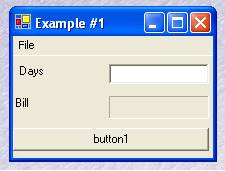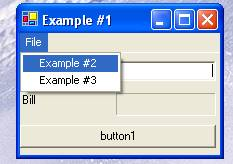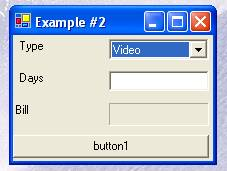# Implementing Inheritance in C#

Overview

Inheritance is one of the most important characteristic of OOP. In this article, I will explain how to implement the inheritance in C# by explaining through an example.

Description

Lets suppose we have a video shop, (a Blockbuster shop like), and we decide that we only want to rent Videos. And again, lets suppose that the price to rent the videos is 1 pound for one day, 2,50 for two days, 4,00 pounds for three days, and 1,50 pounds for each days more.

Example #1:public class Bill
{
private const decimal oneDay = 1.00M;
private const decimal twoDays = 2.50M;
private const decimal threeDays = 4.00M;
private const decimal moreDays = 1.50M;
public decimal Total(int days)
{
decimal bill = 0;
while (days > 3)
{
bill += moreDays;
days--;
}
switch (days)
{
case 1:
bill += oneDay;
break;
case 2:
bill += twoDays;
break;
case 3:
bill += threeDays;
break;
}
return bill;
}
}

Now, lets suppose that we want to rent DVD as well, so that the price will be different, but the formula to calculate the bill will be essentially the same

Example #2:and here the code is:

public class TotalBill
{
private const decimal oneDay = 1.00M;
private const decimal twoDays = 2.50M;
private const decimal threeDays = 4.00M;
private const decimal moreDays = 1.50M;
public virtual decimal Total(int days)
{
decimal bill = 0;
while (days > 3)
{
bill += moreDays;
days--;
}
switch (days)
{
case 1:
bill += oneDay;
break;
case 2:
bill += twoDays;
break;
case 3:
bill += threeDays;
break;
}
return bill;
}
}
public class TotalBillDVD : TotalBill
{
private const decimal oneDay = 2.00M;
private const decimal twoDays = 3.50M;
private const decimal threeDays = 5.00M;
private const decimal moreDays = 2.50M;
public override decimal Total(int days)
{
decimal bill = 0;
while (days > 3)
{
bill += moreDays;
days--;
}
switch (days)
{
case 1:
bill += oneDay;
break;
case 2:
bill += twoDays;
break;
case 3:
bill += threeDays;
break;
}
return bill;
}
}

In this case we have to indicate to the compiler that the class TotalBillDVD inherits from TotalBill, and its method total ha sto override on the method in base class.

This is possible in two steps:

1. In the base class we will indicate the method as virtual;
2. In the inherited class we will indicate that the method overrides the base method.

So, if in the future we want to rent other things, like music CDs or others, well we have just to create other classes. Although theres a problem, if we choose that we need to implement some other methods in the base class, and we dont know if we want it to be inherited, well we could have a problem, if we forget to declare the method as virtual.

A better way to manage the inherits issues is trough the abstract classes. Lets see the last example:

public abstract class Payment
{
public abstract decimal Total(int days);

public class Video : Payment
{
private const decimal oneDay = 1.00M;
private const decimal twoDays = 2.50M;
private const decimal threeDays = 4.00M;
private const decimal moreDays = 1.50M;
public override decimal Total(int days)
{
decimal bill = 0;
while (days > 3)
{
bill += moreDays;
days--;
}
switch (days)
{
case 1:
bill += oneDay;
break;
case 2:
bill += twoDays;
break;
case 3:
bill += threeDays;
break;
}
return bill;
}
}
public class DVD : Payment
{
private const decimal oneDay = 2.00M;
private const decimal twoDays = 3.50M;
private const decimal threeDays = 5.00M;
private const decimal moreDays = 2.50M;
public override decimal Total(int days)
{
decimal bill = 0;
while (days > 3)
{
bill += moreDays;
days--;
}
switch (days)
{
case 1:
bill += oneDay;
break;
case 2:
bill += twoDays;
break;
case 3:
bill += threeDays;
break;
}
return bill;
}
}

Recommended Ebook